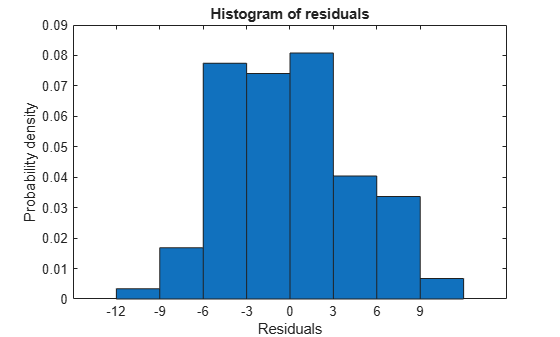## Linear Regression Workflow

This example shows how to fit a linear regression model. A typical workflow involves the following: import data, fit a regression, test its quality, modify it to improve the quality, and share it.

### Step 1. Import the data into a table.

`hospital.xls` is an Excel® spreadsheet containing patient names, sex, age, weight, blood pressure, and dates of treatment in an experimental protocol. First read the data into a table.

`patients = readtable('hospital.xls','ReadRowNames',true);`

Examine the five rows of data.

`patients(1:5,:)`
```ans=5×11 table name sex age wgt smoke sys dia trial1 trial2 trial3 trial4 ____________ _____ ___ ___ _____ ___ ___ ______ ______ ______ ______ YPL-320 {'SMITH' } {'m'} 38 176 1 124 93 18 -99 -99 -99 GLI-532 {'JOHNSON' } {'m'} 43 163 0 109 77 11 13 22 -99 PNI-258 {'WILLIAMS'} {'f'} 38 131 0 125 83 -99 -99 -99 -99 MIJ-579 {'JONES' } {'f'} 40 133 0 117 75 6 12 -99 -99 XLK-030 {'BROWN' } {'f'} 49 119 0 122 80 14 23 -99 -99 ```

The `sex` and `smoke` fields seem to have two choices each. So change these fields to categorical.

```patients.smoke = categorical(patients.smoke,0:1,{'No','Yes'}); patients.sex = categorical(patients.sex);```

### Step 2. Create a fitted model.

Your goal is to model the systolic pressure as a function of a patient's age, weight, sex, and smoking status. Create a linear formula for `'sys'` as a function of `'age'`, `'wgt'`, `'sex'`, and `'smoke'` .

```modelspec = 'sys ~ age + wgt + sex + smoke'; mdl = fitlm(patients,modelspec)```
```mdl = Linear regression model: sys ~ 1 + sex + age + wgt + smoke Estimated Coefficients: Estimate SE tStat pValue _________ ________ ________ __________ (Intercept) 118.28 7.6291 15.504 9.1557e-28 sex_m 0.88162 2.9473 0.29913 0.76549 age 0.08602 0.06731 1.278 0.20438 wgt -0.016685 0.055714 -0.29947 0.76524 smoke_Yes 9.884 1.0406 9.498 1.9546e-15 Number of observations: 100, Error degrees of freedom: 95 Root Mean Squared Error: 4.81 R-squared: 0.508, Adjusted R-Squared: 0.487 F-statistic vs. constant model: 24.5, p-value = 5.99e-14 ```

The sex, age, and weight predictors have rather high $p$-values, indicating that some of these predictors might be unnecessary.

### Step 3. Locate and remove outliers.

See if there are outliers in the data that should be excluded from the fit. Plot the residuals.

`plotResiduals(mdl)`There is one possible outlier, with a value greater than 12. This is probably not truly an outlier. For demonstration, here is how to find and remove it.

Find the outlier.

```outlier = mdl.Residuals.Raw > 12; find(outlier)```
```ans = 84 ```

Remove the outlier.

```mdl = fitlm(patients,modelspec,... 'Exclude',84); mdl.ObservationInfo(84,:)```
```ans=1×4 table Weights Excluded Missing Subset _______ ________ _______ ______ WXM-486 1 true false false ```

Observation 84 is no longer in the model.

### Step 4. Simplify the model.

Try to obtain a simpler model, one with fewer predictors but the same predictive accuracy. `step` looks for a better model by adding or removing one term at a time. Allow `step` take up to 10 steps.

`mdl1 = step(mdl,'NSteps',10)`
```1. Removing wgt, FStat = 4.6001e-05, pValue = 0.9946 2. Removing sex, FStat = 0.063241, pValue = 0.80199 ```
```mdl1 = Linear regression model: sys ~ 1 + age + smoke Estimated Coefficients: Estimate SE tStat pValue ________ ________ ______ __________ (Intercept) 115.11 2.5364 45.383 1.1407e-66 age 0.10782 0.064844 1.6628 0.09962 smoke_Yes 10.054 0.97696 10.291 3.5276e-17 Number of observations: 99, Error degrees of freedom: 96 Root Mean Squared Error: 4.61 R-squared: 0.536, Adjusted R-Squared: 0.526 F-statistic vs. constant model: 55.4, p-value = 1.02e-16 ```

`step` took two steps. This means it could not improve the model further by adding or subtracting a single term.

Plot the effectiveness of the simpler model on the training data.

`plotResiduals(mdl1)`The residuals look about as small as those of the original model.

### Step 5. Predict responses to new data.

Suppose you have four new people, aged 25, 30, 40, and 65, and the first and third smoke. Predict their systolic pressure using `mdl1`.

```ages = [25;30;40;65]; smoker = {'Yes';'No';'Yes';'No'}; systolicnew = feval(mdl1,ages,smoker)```
```systolicnew = 4×1 127.8561 118.3412 129.4734 122.1149 ```

To make predictions, you need only the variables that `mdl1` uses.

### Step 6. Share the model.

You might want others to be able to use your model for prediction. Access the terms in the linear model.

`coefnames = mdl1.CoefficientNames`
```coefnames = 1x3 cell array {'(Intercept)'} {'age'} {'smoke_Yes'} ```

View the model formula.

`mdl1.Formula`
```ans = sys ~ 1 + age + smoke ```

Access the coefficients of the terms.

`coefvals = mdl1.Coefficients(:,1).Estimate`
```coefvals = 3×1 115.1066 0.1078 10.0540 ```

The model is `sys = 115.1066 + 0.1078*age + 10.0540*smoke`, where `smoke` is `1` for a smoker, and `0` otherwise.